# Integral Test Example

Previous: An Integral Test Flowchart

Next: Example: Integral Test with a Logarithm

## Example

Consider the infinite seriesDetermine whether it is convergent using the integral test.

## Complete Solution

### Step 1: Pull Out the Negative Signwhere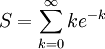If S converges, then the given infinite series converges.

### Step 2: Check to see if the integral test can be applied

Let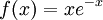. Then

• f(x) is continuous
• f(x) is decreasing
• f(x) is non-negative

Therefore the integral test can be applied.

### Step 3: Apply the Integral Test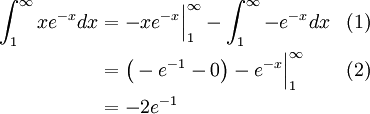### Step 4: Conclusion

The result is finite, so S is convergent by the integral test, so the given series is also convergent.

## Explanation of Each Step

### Step (1)

If we did not pull out the negative sign, we would not be able to apply the integral test, because this test can only be applied when all terms in the series are positive. This simple algebraic manipulation allows us to apply the integral test.

### Step (2)

There are only three criteria we need to check before applying the integral test. Because all three criteria are met, we can apply this test.

### Step (3)

In Step (3) we applied the formula for the integral test, using the method of integration by parts to calculate the integral.

## Possible Challenge Areas

### Algebraic Manipulation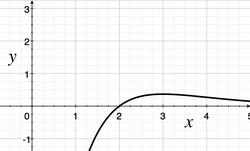If we let a=2, then we see that the function we use to perform the integral test is strictly positive for x>2. Simply pulling out the first few negative terms in the sum that are negative will create a series whose convergence we can test with the integral test.

In the example we were given, we only had to pull out a negative sign, but what if we were asked to determine whether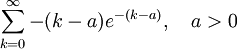converges? How would we approach this problem?

Observing that the function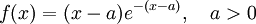is strictly positive for x>a, we can pull out the first terms of the sum that are negative, knowing that the remaining terms are positive, and can be used in the integral test.

Previous: An Integral Test Flowchart

Next: Example: Integral Test with a Logarithm